# Search ResultsCollection Resources(60)

View
Selected filters:
• Trigonometry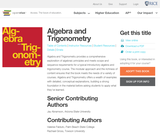Conditions of Use:
No Strings Attached
Rating

Algebra and Trigonometry provides a comprehensive exploration of algebraic principles and meets ...

Algebra and Trigonometry provides a comprehensive exploration of algebraic principles and meets scope and sequence requirements for a typical introductory algebra and trigonometry course. The modular approach and the richness of content ensures that the book meets the needs of a variety of courses. Algebra and Trigonometry offers a wealth of examples with detailed, conceptual explanations, building a strong foundation in the material before asking students to apply what they’ve learned.

Subject:
Algebra
Trigonometry
Material Type:
Textbook
Provider:
Rice University
Provider Set:
OpenStax College
Author:
David Lippman
Jay Abramson
Jean-Marie Magnier
Melonie Rasmussen
Nicholas Belloit
Rachael Gross
Rick Norwood
Valeree Falduto
Date created
3-شوال-1437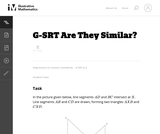Conditions of Use:
Remix and Share
Rating

In this problem, students are given a picture of two triangles that ...

In this problem, students are given a picture of two triangles that appear to be similar, but whose similarity cannot be proven without further information. Asking students to provide a sequence of similarity transformations that maps one triangle to the other focuses them on the work of standard G-SRT.2, using the definition of similarity in terms of similarity transformations.

Subject:
Mathematics
Geometry
Trigonometry
Material Type:
Activity/Lab
Provider:
Illustrative Mathematics
Provider Set:
Illustrative Mathematics
Author:
Illustrative Mathematics
Date created
3-جمادي الأولى-1434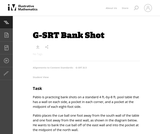Conditions of Use:
Remix and Share
Rating

This task asks students to use similarity to solve a problem in ...

This task asks students to use similarity to solve a problem in a context that will be familiar to many, though most students are accustomed to using intuition rather than geometric reasoning to set up the shot.

Subject:
Mathematics
Geometry
Trigonometry
Material Type:
Activity/Lab
Provider:
Illustrative Mathematics
Provider Set:
Illustrative Mathematics
Author:
Illustrative Mathematics
Date created
3-جمادي الأولى-1434Conditions of Use:
Remix and Share
Rating

This task was developed by high school and postsecondary mathematics and agriculture ...

This task was developed by high school and postsecondary mathematics and agriculture sciences educators, and validated by content experts in the Common Core State Standards in mathematics and the National Career Clusters Knowledge & Skills Statements. It was developed with the purpose of demonstrating how the Common Core and CTE Knowledge & Skills Statements can be integrated into classroom learning - and to provide classroom teachers with a truly authentic task for either mathematics or CTE courses.

Subject:
Geometry
Trigonometry
Material Type:
Activity/Lab
Lesson Plan
Provider:
National Association of State Directors of Career Technical Education Consortium
Provider Set:
Career Technical Education
Date created
7-رمضان-1433Conditions of Use:
Remix and Share
Rating

This task was developed by high school and postsecondary mathematics and design/pre-construction ...

This task was developed by high school and postsecondary mathematics and design/pre-construction educators, and validated by content experts in the Common Core State Standards in mathematics and the National Career Clusters Knowledge & Skills Statements. It was developed with the purpose of demonstrating how the Common Core and CTE Knowledge & Skills Statements can be integrated into classroom learning - and to provide classroom teachers with a truly authentic task for either mathematics or CTE courses.

Subject:
Architecture and Design
Geometry
Trigonometry
Material Type:
Activity/Lab
Assessment
Homework/Assignment
Lesson Plan
Provider:
National Association of State Directors of Career Technical Education Consortium
Provider Set:
Career Technical Education
Date created
13-ربيع الثاني-1433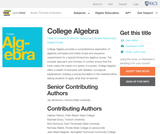Conditions of Use:
No Strings Attached
Rating

College Algebra provides a comprehensive exploration of algebraic principles and meets scope ...

College Algebra provides a comprehensive exploration of algebraic principles and meets scope and sequence requirements for a typical introductory algebra course. The modular approach and richness of content ensure that the book meets the needs of a variety of courses. College Algebra offers a wealth of examples with detailed, conceptual explanations, building a strong foundation in the material before asking students to apply what theyve learned.

Subject:
Algebra
Trigonometry
Material Type:
Textbook
Provider:
Rice University
Provider Set:
OpenStax College
Author:
David Lippman
Jay Abramson
Jean-Marie Magnier
Melonie Rasmussen
Nicholas Belloit
Rachael Gross
Rick Norwood
Valeree Falduto
Date created
4-شوال-1437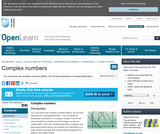Conditions of Use:
Remix and Share
Rating

You may have met complex numbers before, but not had experience in ...

You may have met complex numbers before, but not had experience in manipulating them. This unit gives an accessible introduction to complex numbers, which are very important in science and technology, as well as mathematics. The unit includes definitions, concepts and techniques which will be very helpful and interesting to a wide variety of people with a reasonable background in algebra and trigonometry.

Subject:
Trigonometry
Material Type:
Activity/Lab
Syllabus
Provider:
The Open University
Provider Set:
Open University OpenLearn
Author:
The Open University
Date created
13-ربيع الأول-1432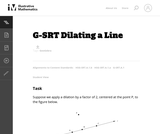Conditions of Use:
Remix and Share
Rating

This task gives students the opportunity to verify that a dilation takes ...

This task gives students the opportunity to verify that a dilation takes a line that does not pass through the center to a line parallel to the original line, and to verify that a dilation of a line segment (whether it passes through the center or not) is longer or shorter by the scale factor.

Subject:
Mathematics
Geometry
Trigonometry
Material Type:
Activity/Lab
Provider:
Illustrative Mathematics
Provider Set:
Illustrative Mathematics
Author:
Illustrative Mathematics
Date created
3-جمادي الأولى-1434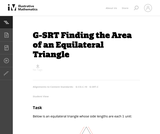Conditions of Use:
Remix and Share
Rating

This tasks examines how to calculate the area of an equilateral triangle ...

This tasks examines how to calculate the area of an equilateral triangle using high school geometry.

Subject:
Mathematics
Geometry
Trigonometry
Material Type:
Activity/Lab
Provider:
Illustrative Mathematics
Provider Set:
Illustrative Mathematics
Author:
Illustrative Mathematics
Date created
3-جمادي الأولى-1434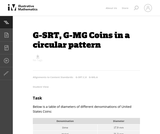Conditions of Use:
Remix and Share
Rating

This task complements ``Seven Circles'' I, II, and III. This is a ...

This task complements ``Seven Circles'' I, II, and III. This is a hands-on activity which students could work on at many different levels and the activity leads to many interesting questions for further investigation.

Subject:
Mathematics
Geometry
Trigonometry
Material Type:
Activity/Lab
Provider:
Illustrative Mathematics
Provider Set:
Illustrative Mathematics
Author:
Illustrative Mathematics
Date created
3-جمادي الأولى-1434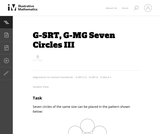Conditions of Use:
Remix and Share
Rating

This task provides an opportunity to model a concrete situation with mathematics. ...

This task provides an opportunity to model a concrete situation with mathematics. Once a representative picture of the situation described in the problem is drawn (the teacher may provide guidance here as necessary), the solution of the task requires an understanding of the definition of the sine function. When the task is complete, new insight is shed on the ``Seven Circles I'' problem which initiated this investigation as is noted at the end of the solution.

Subject:
Mathematics
Geometry
Trigonometry
Material Type:
Activity/Lab
Provider:
Illustrative Mathematics
Provider Set:
Illustrative Mathematics
Author:
Illustrative Mathematics
Date created
3-جمادي الأولى-1434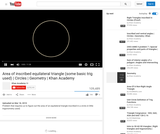Conditions of Use:
Remix and Share
Rating

This geometry lesson demonstrates how to calculate the area of an equilateral ...

This geometry lesson demonstrates how to calculate the area of an equilateral triangle inscribed in a circle and uses a little trigonometry.

Subject:
Geometry
Trigonometry
Material Type:
Lecture
Provider:
Provider Set:
Author:
Khan, Salman
Date created
8-شوال-1432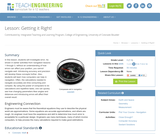Conditions of Use:
Read the Fine Print
Rating

In this lesson, students will investigate error. As shown in earlier activities ...

In this lesson, students will investigate error. As shown in earlier activities from navigation lessons 1 through 3, without an understanding of how errors can affect your position, you cannot navigate well. Introducing accuracy and precision will develop these concepts further. Also, students will learn how computers can help in navigation. Often, the calculations needed to navigate accurately are time consuming and complex. By using the power of computers to do calculations and repetitive tasks, one can quickly see how changing parameters likes angles and distances and introducing errors will affect their overall result.

Subject:
Engineering
Mathematics
Geometry
Trigonometry
Material Type:
Activity/Lab
Lesson Plan
Provider:
TeachEngineering
Provider Set:
TeachEngineering
Author:
Integrated Teaching and Learning Program,
Janet Yowell
Jeff White
Malinda Schaefer Zarske
Matt Lippis
TeachEngineering.org
Date created
13-ربيع الأول-1432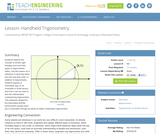Conditions of Use:
Read the Fine Print
Rating

Students explore the concept of similar right triangles and how they apply ...

Students explore the concept of similar right triangles and how they apply to trigonometric ratios. Use this lesson as a refresher of what trig ratios are and how they work. In addition to trigonometry, students explore a clinometer app on an Android® or iOS® device and how it can be used to test the mathematics underpinning trigonometry. This prepares student for the associated activity, during which groups each put a clinometer through its paces to better understand trigonometry.

Subject:
Engineering
Mathematics
Trigonometry
Material Type:
Lesson Plan
Provider:
TeachEngineering
Provider Set:
TeachEngineering
Author:
IMPART RET Program,
Scott Burns
TeachEngineering.org
Date created
14-محرم-1436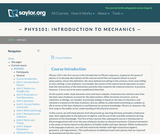Conditions of Use:
Read the Fine Print
Rating

This course will survey physics concepts and their respective applications; it is ...

This course will survey physics concepts and their respective applications; it is intended as a basic introduction to the current physical understanding of our universe. In this course, the student will study physics from the ground up, learning the basic principles of physical law, their application to the behavior of objects, and the use of the scientific method in driving advances in this knowledge. This course focuses on Newtonian mechanics--how objects move and interact--rather than Electromagnetism or Quantum Mechanics. While mathematics is the language of physics, the student need only be familiar with high school-level algebra, geometry, and trigonometry; the small amount of additional math needed will be developed during the course. (Physics 101; See also: Biology 109, Chemistry 001, Mechanical Engineering 005)

Subject:
Trigonometry
Physics
Material Type:
Full Course
Provider:
The Saylor Foundation
Date created
20-ذو الحجة-1432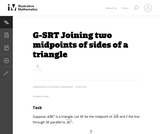Conditions of Use:
Remix and Share
Rating

This task is closely related to very important material about similarity and ...

This task is closely related to very important material about similarity and ratios in geometry.

Subject:
Mathematics
Geometry
Trigonometry
Material Type:
Activity/Lab
Provider:
Illustrative Mathematics
Provider Set:
Illustrative Mathematics
Author:
Illustrative Mathematics
Date created
3-جمادي الأولى-1434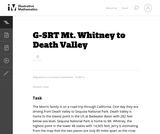Conditions of Use:
Remix and Share
Rating

The purpose of this task is to engage students in an open-ended ...

The purpose of this task is to engage students in an open-ended modeling task that uses similarity of right triangles, and also requires the use of technology.

Subject:
Mathematics
Geometry
Trigonometry
Material Type:
Activity/Lab
Provider:
Illustrative Mathematics
Provider Set:
Illustrative Mathematics
Author:
Illustrative Mathematics
Date created
3-جمادي الأولى-1434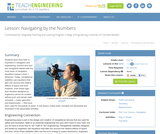Conditions of Use:
Read the Fine Print
Rating

In this lesson, students will learn that math is important in navigation ...

In this lesson, students will learn that math is important in navigation and engineering. Ancient land and sea navigators started with the most basic of navigation equations (Speed x Time = Distance). Today, navigational satellites use equations that take into account the relative effects of space and time. However, even these high-tech wonders cannot be built without pure and simple math concepts basic geometry and trigonometry that have been used for thousands of years. In this lesson, these basic concepts are discussed and illustrated in the associated activities.

Subject:
Engineering
Mathematics
Geometry
Trigonometry
Material Type:
Activity/Lab
Lesson Plan
Provider:
TeachEngineering
Provider Set:
TeachEngineering
Author:
Integrated Teaching and Learning Program,
Janet Yowell
Jeff White
Malinda Schaefer Zarske
TeachEngineering.org
Date created
13-ربيع الأول-1432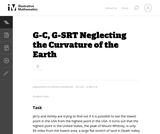Conditions of Use:
Remix and Share
Rating

This task applies geometric concepts, namely properties of tangents to circles and ...

This task applies geometric concepts, namely properties of tangents to circles and of right triangles, in a modeling situation. The key geometric point in this task is to recognize that the line of sight from the mountain top towards the horizon is tangent to the earth. We can then use a right triangle where one leg is tangent to a circle and the other leg is the radius of the circle to investigate this situation.

Subject:
Mathematics
Geometry
Trigonometry
Material Type:
Activity/Lab
Provider:
Illustrative Mathematics
Provider Set:
Illustrative Mathematics
Author:
Illustrative Mathematics
Date created
3-جمادي الأولى-1434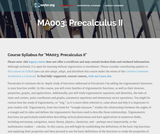Conditions of Use:
Read the Fine Print
Rating

This course begins by establishing the definitions of the basic trig functions ...

This course begins by establishing the definitions of the basic trig functions and exploring their properties, and then proceeds to use the basic definitions of the functions to study the properties of their graphs, including domain and range, and to define the inverses of these functions and establish the their properties. Through the language of transformation, the student will explore the ideas of period and amplitude and learn how these graphical differences relate to algebraic changes in the function formulas. The student will also learn to solve equations, prove identities using the trig functions, and study several applications of these functions. Upon successful completion of this course, the student will be able to: measure angles in degrees and radians, and relate them to arc length; solve problems involving right triangles and unit circles using the definitions of the trigonometric functions; solve problems involving non-right triangles; relate the equation of a trigonometric function to its graph; solve trigonometric equations using inverse trig functions; prove trigonometric identities; solve trig equations involving identities; relate coordinates and equations in Polar form to coordinates and equations in Cartesian form; perform operations with vectors and use them to solve problems; relate equations and graphs in Parametric form to equations and graphs in Cartesian form; link graphical, numeric, and symbolic approaches when interpreting situations and analyzing problems; write clear, correct, and complete solutions to mathematical problems using proper mathematical notation and appropriate language; communicate the difference between an exact and an approximate solution and determine which is more appropriate for a given problem. This free course may be completed online at any time. It has been developed through a partnership with the Washington State Board for Community and Technical Colleges; the Saylor Foundation has modified some WSBCTC materials. (Mathematics 003)

Subject:
Functions
Trigonometry
Material Type:
Full Course
Provider:
The Saylor Foundation
Date created
25-جمادي الأولى-1433